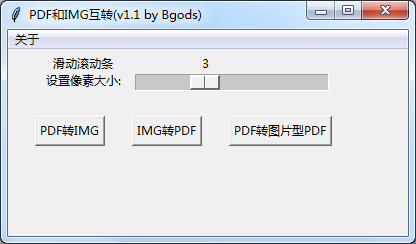## 一、我的测试脚本：

``````def main():
# description参数可以用于描述脚本的参数作用 ...``````

## PDF和图片互转

• PDF转PNG：将pdf转成png图片；
• PNG转PDF：将图片打包成PDF文件；
• PDF转图片型PDF：将文字型PDF转成图片型的PDF。## 利用python遍历多级文件夹处理不同文件

• 需求：近期，同事在处理文件的时候，常常需要从一堆文件中提取一些数据信息；
• 分析：由于每个文件夹下面的文件或文件夹多如牛毛，文件类型也很多种，需要针对不同文件类型做处理，人工处理是不现实的，只能用脚本处理了。

1. 这里定义了一个类 `ErgodicFolder`，里面有两个方法 `process_file``ergodic_path_list`
2. `process_file ...`

## 常用函数

abs
fabs

np.abs()
np.fabs()
sqrt 计算元素的平方根。等价于array ** 0 ...

## 一维数组运算

• 生成一维示例数组
``````>>> a = np.array([1, 2, 3, 4, 5])
>>> b = np.arange(1, 6)
>>> a, b ...``````

## 一、导入 NumPy

• 导入 NumPy

``>>> import numpy as np``
• 查看 NumPy ...

## python3模拟登陆新浪微博

``````import base64
import requests
import re
import rsa
import ...``````

## 递归函数

``````def factorial(n):
if n == 1:
return ...``````

## pandas学习笔记(入门篇)

``import pandas as pd ...``